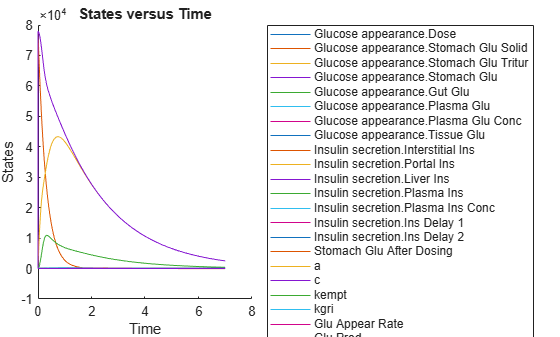# removebyname

Remove simulation data by name from `SimData` object

## Syntax

``[t,x,names] = removebyname(simdata,selectNames)``
``sdOut = removebyname(simdata,selectNames)``
``___ = removebyname(simdata,selectNames,'Format',formatValue)``

## Description

example

````[t,x,names] = removebyname(simdata,selectNames)` returns the simulation time points `t`, the simulation data `x`, and corresponding `names` after removing the simulation data of model components specified by `selectNames`. ```

example

````sdOut = removebyname(simdata,selectNames)` returns the simulation results after removing the simulation data of model components specified by `selectNames` as a `SimData` object `sdOut`. ```

example

````___ = removebyname(simdata,selectNames,'Format',formatValue)` returns the simulation data in the specified data format.```

## Examples

collapse all

Load the model of glucose-insulin response. For details about the model, see the Background section in Simulate the Glucose-Insulin Response.

`sbioloadproject('insulindemo.sbproj','m1');`

Suppress an information warning that is issued during simulations.

`warnSettings = warning('off', 'SimBiology:DimAnalysisNotDone_MatlabFcn_Dimensionless');`

Simulate a single meal for a normal subject for 7 hours.

```singleMeal = sbioselect(m1,'Name','Single Meal'); cs = getconfigset(m1,'active'); cs.StopTime = 7; sd1 = sbiosimulate(m1,singleMeal)```
``` SimBiology Simulation Data ModelName: Cobelli's Glucose-Insulin System Logged Data: Species: 15 Compartment: 0 Parameter: 24 Sensitivity: 0 Observable: 0 ```
`sbioplot(sd1);`Remove all parameter data logged in the `SimData` object sd.

`[t,x,names] = remove(sd1,{'Type','parameter'});`

Remove all parameter data and return as a new `SimData` object.

```sd2 = remove(sd1,{'Type','parameter'}); sbioplot(sd2);```Remove the simulation data of a species by specifying its name.

```sd3 = removebyname(sd2,["[Insulin secretion].[Ins Delay 2]"]); sbioplot(sd3);```Restore the warning settings.

`warning(warnSettings);`

## Input Arguments

collapse all

Simulation data, specified as a `SimData` object or array of `SimData` objects.

Names of states that you want to select data for, specified as a character vector, string, string vector, or cell array of character vectors.

Example: `{'x1','x2','x3'}`

Data Types: `char` | `string` | `cell`

Simulation data format, specified as a character vector or string. Some formats require you to specify only one output argument. The valid formats follow.

• `'num'` — This format returns simulation time points and simulation data in numeric arrays and the names of quantities and sensitivities as a cell array. This format is the default when you run `getdata` with multiple output arguments.

• `'nummetadata'` — This format returns a cell array of metadata structures instead of the names of quantities and sensitivities as the third output argument.

• `'numqualnames'` — This format returns qualified names in the third output argument to resolve ambiguities.

You must specify only one output argument for the following formats.

• `'simdata'` — This format returns data in a new `SimData` object or an array of `SimData` objects. This format is the default when you specify a single output argument.

• `'struct'` — This format returns a structure or structure array that contains both data and metadata.

• `'ts'` — This format returns data as a cell array.

• If `simdata` is scalar, the cell array is an m-by-1 array, where each element is a `timeseries` object. m is the number of quantities and sensitivities logged during the simulation.

• If `simdata` is not scalar, the cell array is k-by-1, where each element of the cell array is an m-by-1 cell array of `timeseries` objects. k is the size of `simdata`, and m is the number of quantities or sensitivities in each `SimData` object in `simdata`. In other words, the function returns an individual time series for each state or column and for each `SimData` object in `simdata`.

• `'tslumped'` — This format returns the data as a cell array of `timeseries` objects, combining data from each `SimData` object into a single time series.

## Output Arguments

collapse all

Simulation time points, returned as a numeric vector or cell array. If `simdata` is scalar, `t` is an n-by-1 vector, where n is the number of time points. If `simdata` is an array of objects, `t` is a k-by-1 cell array, where k is the size of `simdata`.

Simulation data, returned as a numeric matrix or cell array. If `simdata` is scalar, `x` is an n-by-m matrix, where n is the number of time points and m is the number of quantities and sensitivities logged during the simulation. If `simdata` is an array of objects, `x` is a k-by-1 cell array, where k is the size of `simdata`.

Names of quantities and sensitivities logged during the simulation, returned as a cell array. If `simdata` is scalar, `names` is an m-by-1 cell array. If `simdata` is an array of objects, `names` is a k-by-1 cell array, where k is the size of `simdata`.

Simulation results, returned as a `SimData` object.

## Version History

Introduced in R2020a